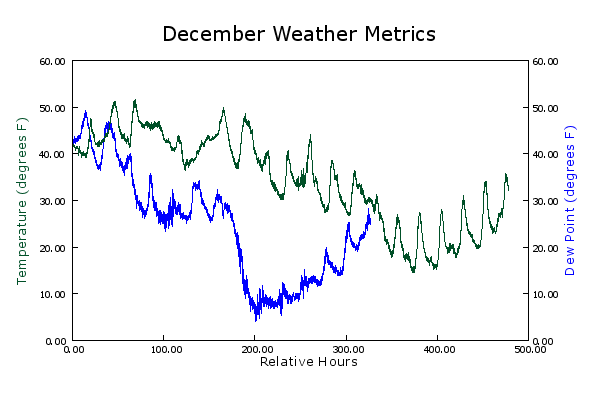simplot
Simplot is a 2-dimensional data plotting application for mathematics and scientific data with a simple interface.

Simplot will make graphs that look like this:using a command line interface that looks like this:

(let ((grn '(0 80 40)) (the-view (mk-view :view-bounds '(0 0 500 60)))) (plot2 "December Weather Metrics" *hour-temp* the-view grn :left "Temperature (degrees F)" grn :bottom "Relative Hours" *time-dewp* the-view +blu+ :right "Dew Point (degrees F)" +blu+))

Simplot depends on the CL-GD package.